# Test: Filters

## 20 Questions MCQ Test Power Electronics | Test: Filters

Description
Attempt Test: Filters | 20 questions in 20 minutes | Mock test for Electrical Engineering (EE) preparation | Free important questions MCQ to study Power Electronics for Electrical Engineering (EE) Exam | Download free PDF with solutions
QUESTION: 1

### In practice the output from the diode rectifier has

Solution:

The output contents along with the DC components the AC harmonics which does no useful work & reduces the efficiency.

QUESTION: 2

### Choose the correct statement

Solution:

A rectifier is used to convert AC to DC. Lower the AC (Non-DC) components in the output lower are the ohmic losses.

QUESTION: 3

### An L filter is connected ________

Solution:

Inductor (L) has a very important property that the current through it cannot change rapidly. We can make use of this property by connecting it in series.

QUESTION: 4

In case of an L filter connected with a rectifier in series with the load, it offers ________ impedance to ac whereas _______ resistance to dc respectively.

Solution:

It offers high impedance to AC such as the AC ripples do not pass through the load.

QUESTION: 5

In case of a C filter, the AC is not allowed to pass to the load by

Solution:

AC ripples are not allowed to pass, by S.C the AC ripples as the C is always connected in parallel with the load.

QUESTION: 6

A capacitor filter or C filter can be used in a rectifier by connecting it

Solution:

AC ripples are not allowed to pass, by S.C the AC ripples as the C is always connected in parallel with the load.

QUESTION: 7

Examine the below shown circuit.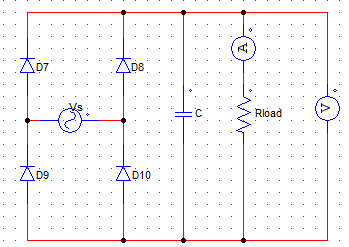While D7 & D10 are reversed biased the

Solution:

When the two are reversed biased and the other two SCR’s are not yet gated, the stored energy in the capacitor is supply the load R.

QUESTION: 8

When a certain type of filter is connected across the R load of a full wave bridge type diode biased rectifier, the following output voltage waveform is obtained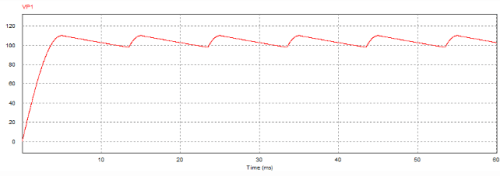The filter connected is most likely to be a/an

Solution:

It is to be noted that the waveform is that of a voltage parameter. The voltage goes up for a very small time and than goes down (discharges) for longer time. For every half wave the peak of the waveform is to the left, if it were to be on the right it would be more likely to be a L filter.

QUESTION: 9

For the below given circuit,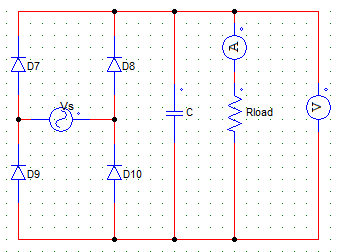When the output current rises, the capacitor current

Solution:

When the load current is increasing, the capacitor is charging through the source voltage & its current keeps on falling & voltage rises.

QUESTION: 10

A single-phase diode B-2 rectifier is fed from a 250 V, 50 Hz source & connected to a load of R = 400 Ω.
Design a capacitor filter such that the ripple factor of the output voltage is less than 5 %. Find the value of the capacitance of the C filter.

Solution:

Use,
C = K * [1 + 1/√2RF] Where, K = 1/4fR.

QUESTION: 11

A diode rectifier with a C filter will have average output voltage _______ than that obtained by a rectifier without the C filter.

Solution:

C filter improves the efficiency and eliminates ripples by keeping the output voltage constant.

QUESTION: 12

An inductor filter connected in series with a resistive load provides a

Solution:

Filter is always connected to the load side (output). Inductor has the property of keeping the current smooth and constant.

QUESTION: 13

In the below given configuration, L is connected as a filter across the R load.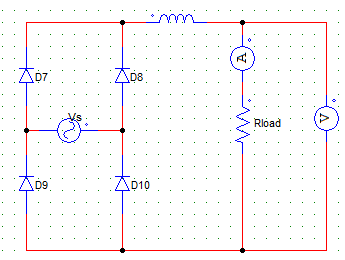The average ammeter current is

Solution:

: I = Vo/R
Vo = 2Vm/π.

QUESTION: 14

The current ripple factor (CRF) is the ratio of

Solution:

CRF = Ir/Io.

QUESTION: 15

In case of an L filter, the ripple current increases with

Solution:

When the load is reduced the resistance R is increased & ripple current increases.

QUESTION: 16

In case of a C filter, if R (load resistance) is increased

Solution:

The time constant RC is increased therefore the ripple factor is reduced.

QUESTION: 17

When a certain type of filter is connected across the R load, of a full wave bridge diode biased rectifier the following output current waveform is obtained.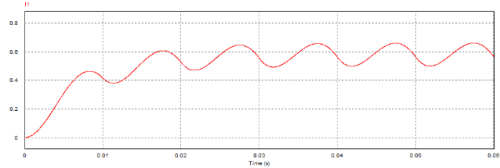The filter connected is most likely a

Solution:

The above waveform is that of the output current. The peak per half cycle is on the right, hence it is more likely to be an L filter.

QUESTION: 18

C filters are suitable for ___________ load resistances and L filters are suitable for _____________ load resistances.

Solution:

In case of L filter, if R is lowered, the time constant (L/R) increases, therefore ripple factor reduces. In case of a C filter, the time constant RC is increased, therefore, ripple factor is reduced.

QUESTION: 19

An LC filter will have ripple factor value___________ (For the same value of L & C)

Solution:

Simply, an LC filter combines both the advantages of L and C filters.

QUESTION: 20

In a single-phase full wave rectifier ___________ order harmonics are the most dominant

Solution:

The output voltage is given by,
Vo = (2Vm/π) – (4Vm/3π)cos 2ωt – (4Vm/15π)cos 4ωt . . . .
Hence the other values after the second factor (4Vm/3π) become very very small hence they can be eliminated.Use Code STAYHOME200 and get INR 200 additional OFF Use Coupon Code